# Routing First-Step: Classless Internet addressing

## Routing and Internet addressing are two important concepts involved in networking. Routing First-Step covers the basics of routing in clear, easy-to-understand language.

The following is the sixth installment of a multi-part series on the fundamentals of routing. Each tip is excerpted from Routing First-Step by William Parkhurst, published by Cisco Press. Check back frequently for the next installment, or go to the main series page for all the installments.

Using a classful IP addressing format worked well when the Internet was relatively small. But as the number of networks on the Internet grew, the limitations of classful addresses became apparent. The Class A address space contains only 125 usable networks in the range 0–127 because networks 0 and 127 are reserved, and network 10 is used for private addressing. Each of these 125 Class A networks could theoretically contain 224 – 2 or 16,777,214 hosts, but it's not realistic to have more than 16 million hosts on the same network. Therefore, in the early 1990s, the Internet moved away from a classful address space to a classless address space. In other words, the number of bits used for the network portion of an IP address became variable instead of fixed.

The network portion of classful IP addresses is fixed. For the network portion of an IP address, Class A addresses use 8 bits, Class B addresses use 16 bits, and Class C addresses use 24 bits. A router could determine the address class by inspecting the first byte of the address. A value of 1–126 is Class A, 128–191 is Class B, and 192–223 is Class C.

For classless IP addressing, there is no longer a relationship between the number of bits used in the network portion and the value of the first byte of the address. A different method has to be used to determine the size of the network portion of an IP address. This new method allows you to borrow bits that are normally used for the host portion of an IP address, and use them to extend the network portion of an IP address.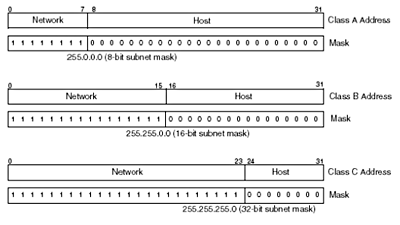There are two common ways to refer to the mask that is used to determine the number of bits used for the network component of an IP address. The first is to use the number of 1 bits in the mask. A Class A mask is an 8-bit mask, Class B is a 16- bit mask, and Class C is a 24-bit mask. The other way is to represent the mask as / (slash) and then the number of 1 bits in the mask. Class A is /8 (slash 8), Class B is /16 (slash 16), and Class C is /24 (slash 24). An important rule is that the number of 1s and 0s in a mask must be contiguous (all the 1s must be together and all the 0s must be together). For example:

11111111 11111111 00000000 00000000 is a valid mask.

11111111 00111111 00000000 00000111 is not a valid mask.

Using a mask to determine the network component of an IP address is called a bitwise logical AND operation. Bitwise AND is equivalent to bitwise multiplication:

A * 1 = A

A * 0 = 0 where A = 0 or 1

A router can determine the network component of the classful IP address 156.26.32.1 by using a mask as shown:

156.26.32.1

AND

255.255.0.0

Equals

156.26.0.0

This might seem like a trivial operation. For classful addresses, this is a fair statement because the network component is on an easy-to-use byte boundary. But you want to be able to switch from classful to classless addressing, and you will need a mask to do that.

As an introduction to classless addressing, assume that your company has been assigned the Class B address 156.26.0.0. If you use this as a classful address, you can have one network with 216-2 or 65,534 hosts. You would like to have more than one network with fewer hosts on each network. This means you will have to create subnets from the assigned Class B address space. Instead of using a 16-bit mask, or /16, see what happens if you use a 17-bit subnet mask:

The Class B part, or 156.26, is fixed and cannot be changed. But your company owns the following 16 bits, so they can be any value you want. The seventeenth bit of your network address can either be a 0 or a 1. If it is 0, that identifies network 156.26.0.0. If the seventeenth bit is a 1, that identifies network 156.26.128.0. By borrowing 1 bit from the standard host portion of the IP address and assigning it to the network portion, you have created two subnets of the Class B address space 156.26. The first subnetwork has host addresses in the range 156.26.0.1– 156.26.127.254.

The second subnetwork has host addresses in the range 156.26.128.1– 156.26.255.254.

This operation is shown in Figure 3-20.

Figure 3-20 - Subnetting a Class B Address with a 17-bit Mask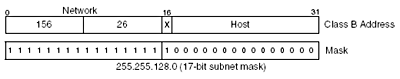If you use 2 additional bits, or a /18 bit mask, you will have four subnets. These four subnets are identified by the four values possible with 2 bits:

0 0

0 1

1 0

1 1

Remember, the network is identified by setting the host portion of the IP address to 0. So, the first subnet using an 18-bit mask is 156.26.0.0.

The second subnet is determined by calculating the value of the third byte when the most significant bits are 0 1:

0 1 0 0 0 0 0 0 = 64

Subnet 2 has a network address of 156.26.64.0.

The third subnet is determined by calculating the value of the third byte when the most significant bits are 1 0:

1 0 0 0 0 0 0 0 = 128

Subnet 2 has a network address of 156.26.128.0.

And the fourth subnet is determined by calculating the value of the third byte when the most significant bits are 1 1:

1 1 0 0 0 0 0 0 = 192

Subnet 4 has a network address of 156.26.192.0.

If you continue this logic, you obtain the information in Table 3-12.

Table 3-12 - Number of Networks and Hosts for a Subnetted Class B Network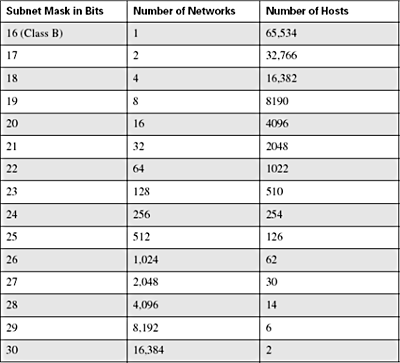The first entry is for a Class B network. Increase the subnet mask by 1 bit, and calculate the number of subnetworks and hosts to find the remaining entries. The number of possible subnetworks is 2 raised to the power of the number of extra bits used for the network. The number of hosts is 2 to the power of the bits left over for the host portion of the address – 2 (broadcast and "this" network addresses).

You do not have to use only one subnet mask to divide the 156.26.0.0 address space in subnetworks. You can use different masks on different networks. For example, assume you have the following requirements for your company's network:

• A maximum of 60 Class C size networks (1–254 hosts)
• A maximum of 14 networks having a maximum of 10 hosts
• Four point-to-point networks

You need to satisfy these requirements, and you want to have addresses in reserve that you can use if your company expands. Where do you start? There is not just one correct way of doing this. You have a Class B address space assigned to you, and you shall see that this will not be that difficult. First, let's subnet the Class B address space into four equal size pieces. For four subnets, you will need to use 2 bits from the host address or a /18 subnet mask. The third byte of the IP address is divided as

N N H H H H H H (2 bits for the network and 6 bits for the host)

To determine the network numbers, first set the host bits to 0:

N N 0 0 0 0 0 0

The possible network values for the third byte are

0 0 0 0 0 0 0 0 = 0

0 1 0 0 0 0 0 0 = 64

1 0 0 0 0 0 0 0 = 128

1 1 0 0 0 0 0 0 = 192

The 156.26 address space is now divided into the following networks:

156.26.0.0

156.26.64.0

156.26.128.0

156.26.192.0

To satisfy the first requirement of a maximum of 60 Class C size networks, subnet the 156.26.0.0/18 address into Class C size or /24 subnets. How many Class C size subnets will this provide? We are using an additional 6 bits to subnet the 156.26.0.0/18 network, and 26 = 64 subnets. This will be sufficient to satisfy the first requirement. The Class C networks will have the following addresses:

156.26.0.0/24

156.26.1.0/24

156.26.2.0/24

...

156.26.62.0/24

156.26.63.0/24

How were these network numbers determined? The 156.26.0.0/18 network was derived from the 156.26.0.0/16 network. The first 16 bits are fixed and equal to 156.26. The next 2 bits are fixed and equal to 0 because this is the subnet used for the Class C size networks. Therefore, the possible range of values for the third byte are

0 0 0 0 0 0 0 0 = 0

0 0 0 0 0 0 0 1 = 1

0 0 0 0 0 0 1 0 = 2

...

0 0 1 1 1 1 1 0 = 62

0 0 1 1 1 1 1 1 = 63

For the first requirement, use networks 156.26.0.0/24 through 156.26.59.0/24.

To satisfy the second requirement, use the last Class C size network, 156.26.63.0, and subnet it to the proper size. For a maximum of 10 hosts, you will need 4 bits for the host address. With 4 bits, a network can support 14 hosts (16 – 2). Because a Class C size network is being subnetted, there are only 8 bits to work with (the last byte). Four bits are needed for the hosts, which leaves 4 bits for the network. The requirement is 14 networks, and 4 network bits can support 16 networks. The last byte is divided, so 4 bits are used for the network and 4 bits for the host:

N N N N H H H H

0 0 0 0 0 0 0 0 = 0 156.26.63.0/28

0 0 0 1 0 0 0 0 = 16 156.26.63.16/28

0 0 1 0 0 0 0 0 = 32 156.26.63.32/28

......

1 1 1 0 0 0 0 0 = 224 156.26.63.224/28

1 1 1 1 0 0 0 0 = 240 156.26.63.240/28

156.26.63.1–156.26.63.14

156.26.63.17–156.26.63.30

156.26.63.33–156.26.63.46

...

156.26.63.225–156.26.63.238

156.26.63.241–156.26.63.254

For the final requirement of four point-to-point networks, the 156.26.63.240 network will be subnetted using a 30-bit mask. A point-to-point network requires only two host addresses.

There are 4 bits available on the 156.26.63.240/28 subnet. Two are needed for the host bits. The two remaining bits are sufficient for the four point-to-point networks that are required. The last byte of the 156.26.63.240 is used for the final subnetting operation:

1 1 1 1 N N H H

The network numbers using a 30-bit mask are

1 1 1 1 0 0 0 0 = 240 156.26.63.240

1 1 1 1 0 1 0 0 = 244 156.26.63.244

1 1 1 1 1 0 0 0 = 248 156.26.63.248

1 1 1 1 1 1 0 0 = 252 156.26.63.252

156.26.63.241 and 242

156.26.63.245 and 246

156.26.63.249 and 250

156.26.63.253 and 254

The final plan is shown in Figure 3-21.

Figure 3-21 - Subnetting a Class B Address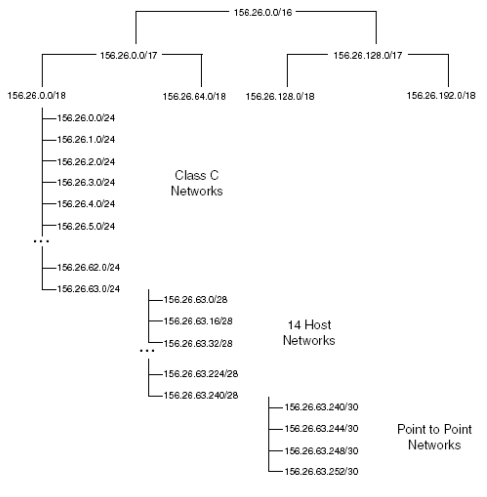If this is your first experience dealing with subnet masks and you find it a bit confusing, take comfort in the fact that this is normal. Subnets and subnet masks take time to master. Get some paper and a pencil and practice, practice, practice. To aid in your understanding, try the following problems:

Answer: Set the 16 host bits to 1 to obtain 156.26.255.255.

Answer: Set the 8 host bits to 1 to obtain 156.26.0.255.

Answer: Set the 4 host bits to 1 to obtain 156.26.0.15.

4. The Class C address 195.14.22.0 is subnetted using a 27-bit subnet mask. How many subnets are there and what are the network numbers?

Answer: The natural mask for a Class C address is /24. Therefore, 33 additional bits are used for the subnet, 23 = 8, so there are eight subnets. The 3 additional network bits are taken from the fourth byte so the network numbers are

0 0 0 0 0 0 0 0 = 0 195.14.22.0/27

0 0 1 0 0 0 0 0 = 32 195.14.22.32/27

0 1 0 0 0 0 0 0 = 64 195.14.22.64/27

0 1 1 0 0 0 0 0 = 96 195.14.22.96/27

1 0 0 0 0 0 0 0 = 128 195.14.22.128/27

1 0 1 0 0 0 0 0 = 160 195.14.22.160/27

1 1 0 0 0 0 0 0 = 192 195.14.22.192/27

1 1 1 0 0 0 0 0 = 224 195.14.22.224/27

5. What is the range of host addresses for the network 195.14.22.64/27?

0 1 0 1 1 1 1 1 = 95 or 195.14.22.95

### IP routing and route summarization

The network in Figure 3-22 is a partial implementation of the addressing plan developed for the 156.26.0.0 network.

Figure 3-22 - Example Network for Route Summarization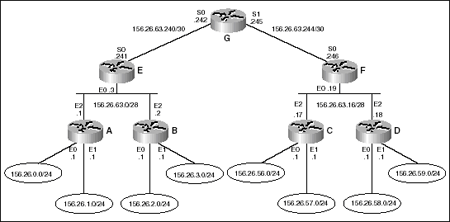Routers A, B, C, and D are access routers and each one connects to two Class C size networks. Routers E and F are the distribution routers, and Router G is the core router. The terminology used in Figure 3-22 is explained in Figure 3-23.

The network in Figure 3-22 has 12 subnets, so each router will have 12 entries in its IP routing table. The routing table for Router G is listed in Table 3-13. Initially, the only routes in the IP routing table are the directly connected networks. The other subnets need to be learned either statically or dynamically. Statically means that every route has to be manually entered on every router. The network has 7 routers so 7 * 12, or 84, routes would need to be entered for IP routing to work. Certainly this can be done, but it would take some time and would be prone to error. Imagine entering all routes statically for a network with hundreds of routers and thousands of routes. This is not a scalable solution. A better solution is to use a dynamic IP routing protocol that will dynamically advertise routes throughout your network. The later chapters will discuss IP routing protocols. For now, assume that all the routes have been entered statically.

Figure 3-23 - Network Terminology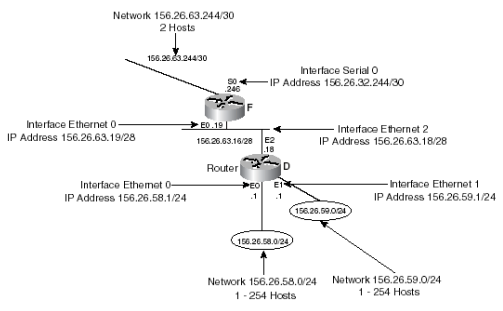Table 3-13 - IP Routing Table for Router G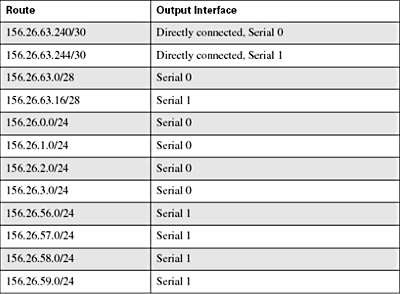The network in Figure 3-22 is similar to the network that was developed in Chapter 1 for the statewide delivery of mail. Router G is equivalent to the core post office that routed mail between states, and between cities in a state. Routers E and F are equivalent to the distribution post offices that routed mail between the access post offices and the state post office. Routers A, B, C, and D are equivalent to the access post offices that routed mail between streets (networks) and the distribution post offices. For the statewide postal network, the core post office did not need to know about every street. It was sufficient to route mail based on the city name. For routing between states, the core post office did not need to know the route to every city and every street in another state. It was sufficient to route interstate mail based on the state name alone. This process of information hiding, or route reduction, was called route summarization or aggregation. It would be nice if IP routes could be aggregated to reduce the size of the routing tables.

Routes are summarized, or aggregated, by reversing the subnetting process. For example, in Figure 3-21, the 156.26.63.240/28 network was subnetted into 4 /30 networks:

156.26.63.240/30

156.26.63.244/30

156.26.63.248/30

156.26.63.252/30

A router can have these four specific routes in the routing table. Or, a router can have one route, or IP prefix, that summarizes these four specific networks. The summary prefix 156.26.63.240/28 contains every possible subnet of 156.26.63.240/28 in the same way that a state contains every possible city and street name contained within that state. The state name summarizes all the city and street names into one prefix. A summary address allowed the core post office to maintain one route to another state and not a route for every possible destination in the other state.

A summary prefix should only summarize those subnets that are actually being used. The prefix 156.26.0.0/16 summarizes all the subnets of the Class B address space 156.26.0.0. So the prefix 156.26.0.0/16 does summarize the four specific /30 subnets of 156.26.63.240/28, but it also summarizes all other subnets of 156.26.0.0/16. This summary tells a router that all subnets of 156.26.0.0/16 are reachable even though many of the subnets might not be in use.

For the network in Figure 3-22 and subnets in Table 3-13, the subnets can be summarized into one route advertisement.

For Router G, 156.26.0.0/24 through 156.26.3.0/24 can be reached through interface serial 0. If you look at the bit patterns of these four subnets, you can determine the subnet mask to use to summarize these routes. It is sufficient, in this case, to examine only the third byte of the subnets:

0 = 0 0 0 0 0 0 0 0

1 = 0 0 0 0 0 0 0 1

2 = 0 0 0 0 0 0 1 0

3 = 0 0 0 0 0 0 1 1

The subnet mask that needs to be used should include only those bits that do not change. For these four routes, the upper 6 bits do not change. These 6 bits need to be included in the summary subnet mask. The value of the mask for the third byte is 1 1 1 1 1 1 0 0 = 252, so the required subnet mask is 255.255.252.0.

Applying the same process to the subnets 156.26.56.0/24 through 156.26.59.0/24, the values of the third byte are

56 = 0 0 1 1 1 0 0 0

57 = 0 0 1 1 1 0 0 1

58 = 0 0 1 1 1 0 1 0

59 = 0 0 1 1 1 0 1 1

As with the previous example, the upper 6 bits need to be included in the subnet mask and the required mask is again 255.255.252.0. The new routing table for Router G is listed in Table 3-14.

Table 3-14 - IP Routing Table for Router G Using Summary Prefixes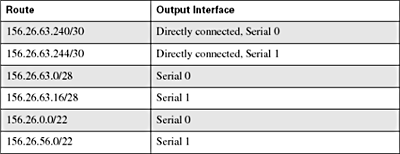The routing table on Router G has been reduced from 12 to 6 routes, a significant reduction. Notice that the two new summary prefixes have a 22-bit subnet mask instead of a 24-bit subnet mask. To see how this works, assume Router G receives a packet for the host at IP address 156.26.2.37. There is no subnet mask information in a destination IP address. The router will find the best match for this route from the routing table. An address with /32 is a host address:

156.26.2.37/32 = 10011100 00011010 00000010 00100101

156.26.0.0/22= 10011100 00011010 00000000 00000000

There is a 22-bit match between the host address and the prefix 156.25.0.0/22, so this packet will be forward using interface serial 0.

What if subnet 156.26.3.0/24 was moved to Router C? (See Figure 3-24.)

Figure 3-24 - Summary and Specific IP Prefixes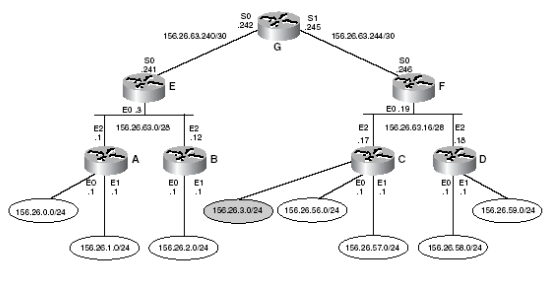Can we still summarize the networks attached to Routers A and B? Yes. The summary prefix 156.26.0.0/22 contains 156.26.0.0/24 through 156.26.3.0/24, so Router G thinks it can reach the 156.26.3.0/24 network through Router E. You can keep this summary prefix as long as a more specific prefix for network 152.26.3.0/24 is added to the routing table on Router G. (See Table 3-15.)

Table 3-15 - IP Routing Table for Router G Using Summary Prefixes and a More Specific Prefix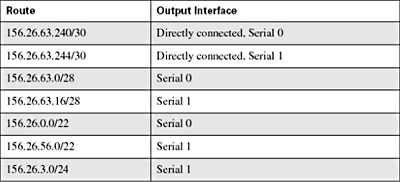Router G now has two routes to subnet 156.26.3.0/24. Which one will it use? Assume Router G receives a packet for host 156.26.3.12/32. Router G will compare this route with the entries in the routing table and there are two that match. This matches 22 bits in the host address:

156.26.0.0/22 = 10011100 00011010 00000000 00000000

156.26.3.12/32 = 10011100 00011010 00000011 00001100

This matches 24 bits and the longest match wins. Router G will forward the packet to Router F:

156.26.3.0/24 = 10011100 00011010 00000011 00000000

156.26.3.12/32 = 10011100 00011010 00000011 00001100

Try reinforcing the key points with the following questions:

1. How many subnets of the Class C address 197.45.120.0/24 are there that can support at least 12 hosts?

Answer: Four bits are required for 12 hosts (24 – 2 = 14). This is a Class C address, so there are 4 bits left for the network. Therefore, there are 16 subnets that can support at least 12 hosts.

2. What are the network numbers for the subnets in the previous question?

Answer: The first 4 bits of the last byte are included in the network number.

0 0 0 0 0 0 0 0 = 0 197.45.120.0

0 0 0 1 0 0 0 0 = 16 197.45.120.16

0 0 1 0 0 0 0 0 = 32 197.45.120.32

0 0 1 1 0 0 0 0 = 48 197.45.120.48

0 1 0 0 0 0 0 0 = 64 197.45.120.64

0 1 0 1 0 0 0 0 = 80 197.45.120.80

0 1 1 0 0 0 0 0 = 96 197.45.120.96

0 1 1 1 0 0 0 0 = 112 197.45.120.112

1 0 0 0 0 0 0 0 = 128 197.45.120.128

1 0 0 1 0 0 0 0 = 144 197.45.120.144

1 0 1 0 0 0 0 0 = 160 197.45.120.160

1 0 1 1 0 0 0 0 = 176 197.45.120.176

1 1 0 0 0 0 0 0 = 192 197.45.120.192

1 1 0 1 0 0 0 0 = 208 197.45.120.208

1 1 1 0 0 0 0 0 = 224 197.45.120.224

1 1 1 1 0 0 0 0 = 240 197.45.120.240

3. Summarize the 16 networks from the previous example into two equal size prefixes.

Answer: Examine the bit patterns of the fourth byte of the first 8 subnets.

0 0 0 0 0 0 0 0 = 0 197.45.120.0

0 0 0 1 0 0 0 0 = 16 197.45.120.16

0 0 1 0 0 0 0 0 = 32 197.45.120.32

0 0 1 1 0 0 0 0 = 48 197.45.120.48

0 1 0 0 0 0 0 0 = 64 197.45.120.64

0 1 0 1 0 0 0 0 = 80 197.45.120.80

0 1 1 0 0 0 0 0 = 96 197.45.120.96

0 1 1 1 0 0 0 0 = 112 197.45.120.112

The only bit that is constant is the first bit, so a 25-bit mask is needed. The summary for the first eight subnets is

197.45.120.0/25

The only bit that is constant for the second set of eight subnets is again the first bit and it is always 1. The summary for the second set of eight subnets is

197.45.120.128/25.

### Supernets

When more bits are used than the natural mask length for the network portion of a Class A, B, or C address, this process was called subnetting. The natural mask for a Class A address is 8 bits. If more than 8 bits are used for the network portion of the IP address, we say that the Class A address has been subnetted.

You can also use fewer bits than the natural mask for the network portion. This process is called supernetting. For example, assume your company owns the following four Class C addresses:

200.10.4.0/24

200.10.5.0/24

200.10.6.0/24

200.10.7.0/24

You can aggregate the addresses using a 22-bit mask, which is 2 bits less than the natural 24-bit mask. The process is the same as subnetting, but the term that is used depends on whether more or fewer bits than the natural mask are being used. The supernet for these networks is 200.10.4.0/22.

All parts reproduced from the book Routing First-Step, ISBN 1587201224, Copyright 2005, Cisco Systems, Inc. Reproduced by permission of Pearson Education, Inc., 800 East 96th Street, Indianapolis, IN 46240. Written permission from Pearson Education, Inc. is required for all other uses. Visit www.ciscopress.com for a detailed description and to learn how to purchase this title.